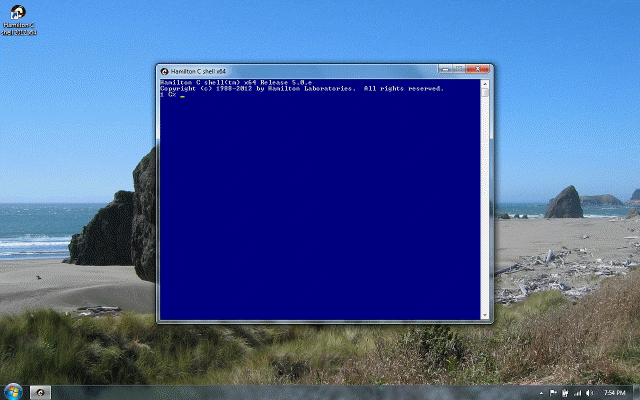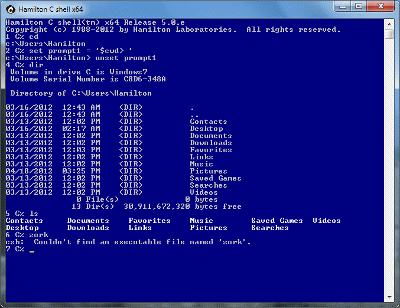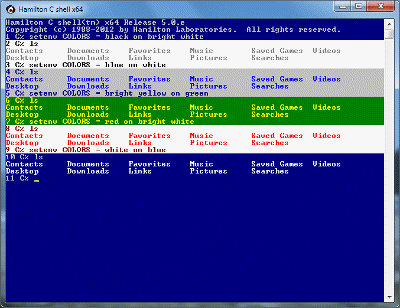## Expression operators### Expression operatorsPrevious | Next

#### Syntax

In order of decreasing precedence.

Operator Meaning
`(   )`
Grouping or procedure call arguments
`{   }`
Run the enclosed statement list and return 1 if it succeeds or 0 otherwise.
`[   ]`
Array indexing. (The first element is element 0.)
```-A   -C   -D   -H
-R   -S   -d   -e
-f   -o   -w   -x
-z   -t   -s```

File system tests. Each takes a single word which may contain wildcards as an operand.

`++   --`
Prefix and postfix increment/decrement. Right to left associativity.
`~    -    !    +`
Bitwise, arithmetic and logical complements and unary plus. Right to left associativity.
** Exponentiation. Right to left associativity.
`*    /    %    //`
Multiplication, division, remainder and integer division
`+    -`
`<<   >>`
Bit-shifting
`<    <=   >=   >`
Relation testing operators
`==   !=   =~   !~`
Equality and pattern-matching operators
& Bit And
^ Bit Xor
| Bit Or
&& Logical And
|| Logical Or
`?    :`
Conditional selection. Right to left associativity.
```=    +=   -=   *=
/=   %=   //=  >>=
<<=  &=   ^=   |=
**=```
Assignment operators

#### Usage

Precedence and associativity is the same as C except for the addition of the new operators. Except as noted, operators of equal precedence group left to right so, e.g., 10/5*2 is read as (10/5)*2 = 4, not 10/(5*2) = 1.

Expressions result in sensible types, considering both the types and the values of the operands. For example, 10/2 returns the integer 5 but 5/2 produces the floating point value 2.5. Also, the integer 1, the floating point value 1.0 and the string "1" all compare equal.

### Previous | NextHamilton C shell, as it first wakes up.A first few commands.You can set the screen colors to your taste.